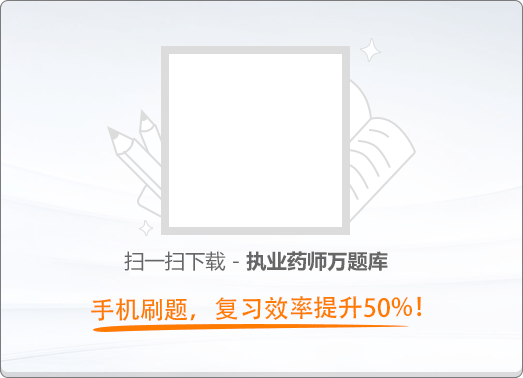90:00《药事管理与法规》历年真题试卷（三）

1
(单项选择题)

• A.

公共卫生服务体系

• B.

医疗服务体系

• C.

医疗保障体系

• D.

药品供应保障体系

• E.

医疗卫生人才体系

• A
• B
• C
• D
• E

2
(单项选择题)

• A.

安全性

• B.

经济性

• C.

稳定性

• D.

均一性

• E.

有效性

• A
• B
• C
• D
• E

4
(单项选择题)

《中华人民共和国行政处罚法》规定，对当事人可不予行政处罚的情形是（　）。

• A.

受他人胁迫有违法行为的

• B.

主动消除或者减轻违法行为危害后果的

• C.

配合行政机关查处违法行为有立功表现的

• D.

违法行为轻微并及时纠正，没有造成危害后果的

• E.

间歇性精神病人在精神正常时有违法行为的

• A
• B
• C
• D
• E

7
(单项选择题)

• A.

先向国家食品药品监督管理总局递交申请，批准后方可生产

• B.

是市场短缺的药品品种

• C.

经国务院或省级药品监督管理部门批准，在指定的医疗机构之间调剂使用

• D.

经省级药品检验所检验合格后供患者使用

• E.

在突发重大疫情时通过零售药店销售

• A
• B
• C
• D
• E

8
(单项选择题)

• A.

具有依法经过资格认定的药学技术人员

• B.

具有与所经营药品相适应的营业场所、设备、仓储设施、卫生环境

• C.

具有保证所经营药品质量管理的规章制度

• D.

具有能对所经营药品进行质量检验的机构

• E.

具有与所经营品种相适应的质量管理机构或人员

• A
• B
• C
• D
• E

10
(单项选择题)

• A.

说明药品的适应症和功能主治

• B.

利用患者介绍药品的作用

• C.

资助电视健康节目并在期间做间歇的宣传

• D.

宣传与某大学的研究机构合作研究开发

• E.

利用某演员做宣传

• A
• B
• C
• D
• E

12
(单项选择题)

《中华人民共和国药品管理法实施条例》规定，中药饮片包装必须印有或者贴有（　）。

• A.

标签

• B.

拉丁文名称

• C.

中药饮片标识

• D.

功能与主治

• E.

禁忌

• A
• B
• C
• D
• E

13
(单项选择题)

• A.

在限定条件下可以依法批准进口

• B.

不允许进口

• C.

经出口国或地区药品管理部门批准后可以进口

• D.

只要有市场就可以进口

• E.

可无条件进口

• A
• B
• C
• D
• E

14
(单项选择题)

• A.

省级卫生行政部门

• B.

省级药品监督管理部门

• C.

国务院卫生行政部门

• D.

地市级药品监督管理部门

• E.

国家药品监督管理部门

• A
• B
• C
• D
• E

15
(单项选择题)

• A.

应当经国家药品监督管理局批准

• B.

可以自行向邻省的医疗机构供应第一类精神药品以便满足边远地区的需求

• C.

应当经所在地卫生行政部门批准，向本省内销售第一类精神药品

• D.

可以向科研单位提供麻醉药品和第一类精神药品小包装原料药

• E.

应当在申请定资格前，2年内没有违反有关禁毒的法律、行政法规规定的行为

• A
• B
• C
• D
• E

16
(单项选择题)

• A.

医疗机构法定代表人的变更

• B.

医疗管理部门负责人的变更

• C.

药剂科主任的变更

• D.

具有麻醉药品处方审核资格的药师的变更

• E.

麻醉药品采购人员的变更

• A
• B
• C
• D
• E

20
(单项选择题)

• A.

安全性评估结果

• B.

药物经济学

• C.

临床药理学

• D.

药品通用名称

• E.

临床治疗首选程度

• A
• B
• C
• D
• E

21
(单项选择题)

• A.

药品的适用性

• B.

药品的稳定性

• C.

药品的可靠性

• D.

药品的安全性

• E.

药品的有效性

• A
• B
• C
• D
• E

22
(单项选择题)

• A.

标签和内包装

• B.

说明书和大包装

• C.

标签和说明书

• D.

内包装和大包装

• E.

标签和大包装

• A
• B
• C
• D
• E

23
(单项选择题)

• A.

药品金额

• B.

临床诊断

• C.

药品名称

• D.

药品性状

• E.

用法用量

• A
• B
• C
• D
• E

24
(单项选择题)

• A.

中药生产基地、药品研发基地、疾控中心

• B.

乡镇卫生院、药品经营企业、药品检验机构

• C.

药品生产企业、药品经营企业、药物临床前研究基地

• D.

药品批发企业、医疗门诊部、新药研发机构

• E.

医疗机构、药品经营企业、药品生产企业

• A
• B
• C
• D
• E

25
(单项选择题)

• A.

药品改变剂型

• B.

药品改变给药途径

• C.

药品改变适应症

• D.

药品在原申请范围内补充说明的

• E.

生产已批准上市的已有国家标准的药品

• A
• B
• C
• D
• E

27
(单项选择题)

• A.

国家食品药品监督管理总局

• B.

疫苗销售地省级药品监督管理部门

• C.

乙药品生产商

• D.

甲药品批发企业

• E.

甲药品批发企业所在地省级药品监督管理部门

• A
• B
• C
• D
• E

28
(单项选择题)

• A.

《药品经营许可证》有效期届满未换证的

• B.

药品经营企业终止经营药品或者关闭的

• C.

《药品经营许可证》被依法撤销、撤回、吊销、收回、缴销或者宣布无效的

• D.

违法药品广告规定的

• E.

不可抗力导致《药品经营许可证》的许可事项无法实施的

• A
• B
• C
• D
• E

30
(单项选择题)

• A.

销售给经营企业

• B.

在医院的网站进行广告宣传

• C.

通过互联网销售制剂

• D.

将制剂的价格与其他药品一起公示

• E.

给在异地的患者，通过邮寄少量制剂

• A
• B
• C
• D
• E

31
(单项选择题)

• A.

具有与设立药品批发企业一致的条件

• B.

对上网交易的品种有完整的管理制度与措施

• C.

具有完整保存交易记录的能力、设施和设备

• D.

具有与上网交易的品种相适应的药品配送系统

• E.

具有负责网上实时咨询的执业药师

• A
• B
• C
• D
• E

32
(单项选择题)

• A.

市场上已有供应，但价格昂贵的品种

• B.

市场上没有供应的经典方剂

• C.

中药、化学药组成的复方制剂

• D.

市场上供应不足的生物制品

• E.

市场上没有供应的中药注射剂

• A
• B
• C
• D
• E

35
(单项选择题)

• A.

有效期至2012年06月

• B.

有效期至2012/06

• C.

有效期至2012.6.6

• D.

有效期至2012.06.06

• E.

有效期至2012年06月06日

• A
• B
• C
• D
• E

38
(单项选择题)

• A.

药品监督管理部门

• B.

物价管理部门

• C.

工商行政管理部门

• D.

卫生行政管理部门

• E.

公安部门

• A
• B
• C
• D
• E

39
(单项选择题)

《互联网药品信息服务管理办法》规定，提供互联网药品信息服务的网站可以发布信息的产品有（　）。

• A.

处方药

• B.

第一类精神药品

• C.

第二类精神药品

• D.

戒毒药品

• E.

医疗用毒性药品

• A
• B
• C
• D
• E

40
(单项选择题)

• A.

要求经营者提供商品的成分、生产工艺、有效期限

• B.

获得质量保障、价格合理、计量正确等公平交易条件

• C.

使用商品受到人身、财产损害的，可以要求经营者或生产者赔偿

• D.

依法成立维护自身合法权益的社会团体

• E.

对经营者提供的商品进行比较、鉴别和监督

• A
• B
• C
• D
• E